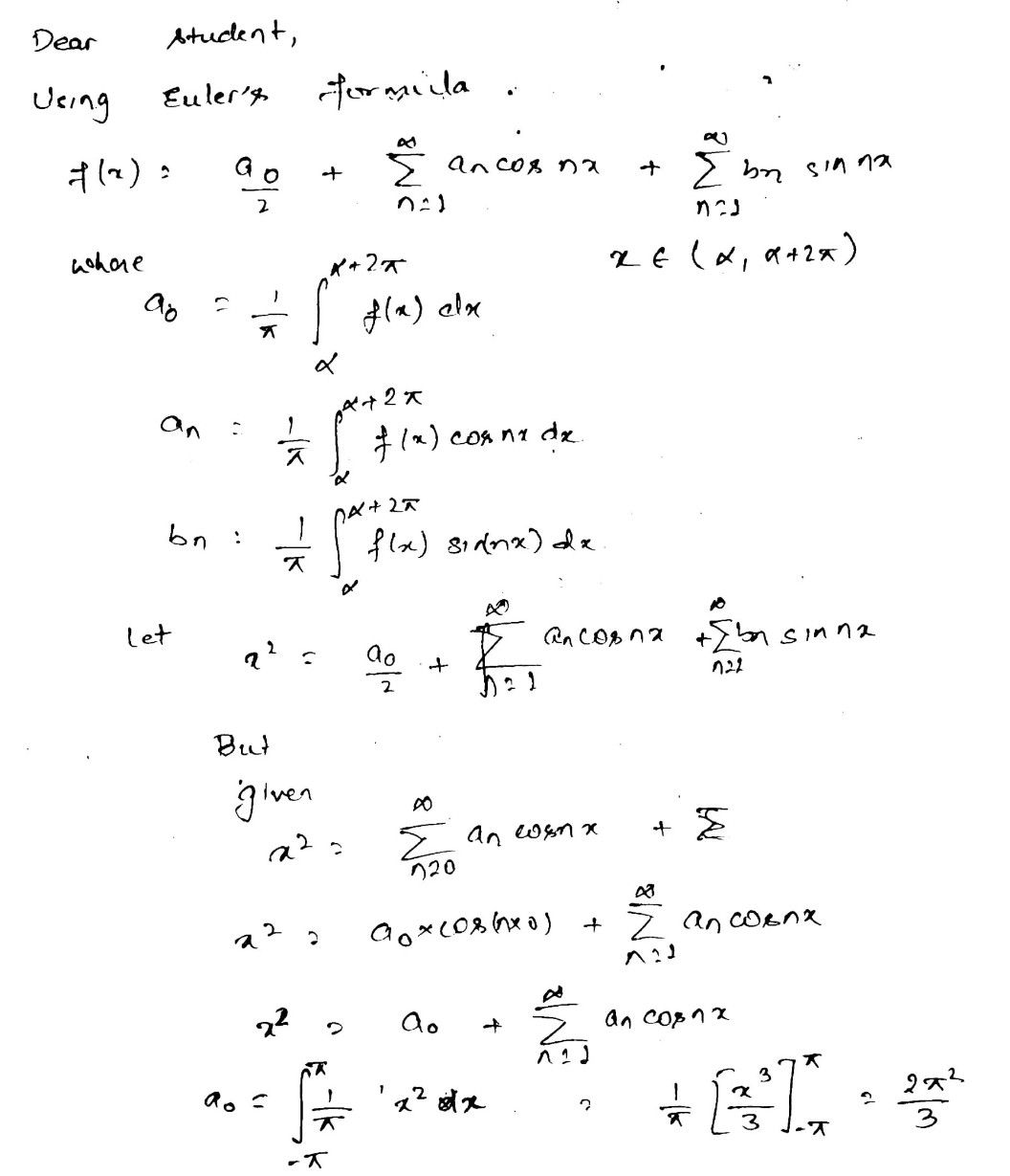Symbol
ProblemfR R, $f\left(x\right)=x^{2},-\pi \leq x\leq \pi$ and $\left(x+2\pi \right)=f\left(x\right)$ VrER. Ifthe fourier series of f(x) is represented as S $x\right)=\sum$ a, cos nx, then a